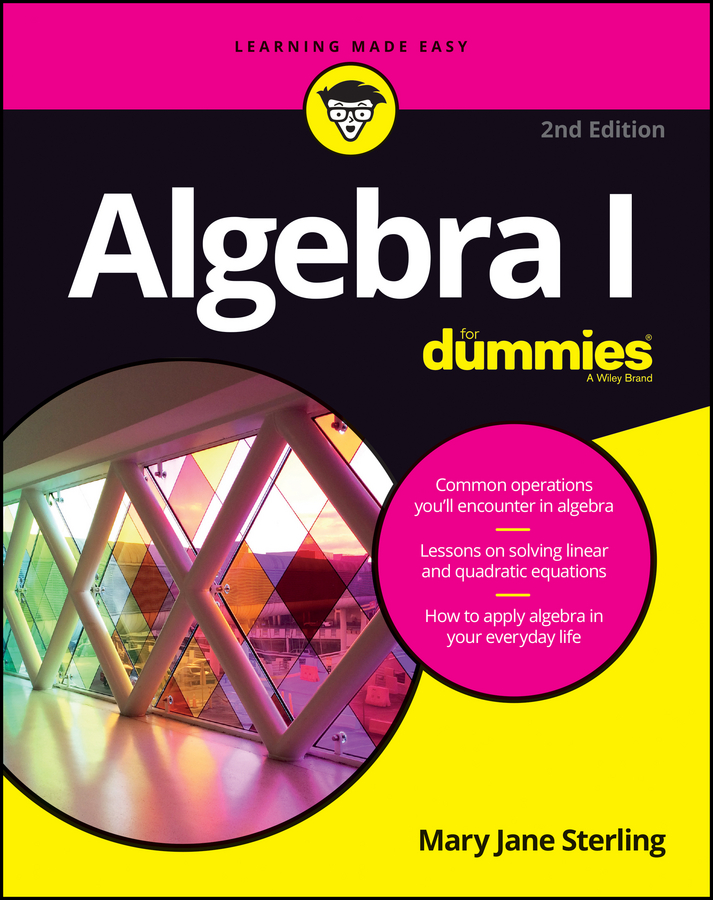##### Algebra I For DummiesDistributing items is an act of spreading them out equally. Algebraic distribution means to multiply each of the terms within the parentheses by another term that is outside the parentheses. To distribute a term over several other terms, you multiply each of the other terms by the first term.

Distribution involves multiplying each individual term in a grouped series of terms by a term outside of the grouping.

a(b + c) = a × b + a × c

a(bc) = a × ba × c

A term is made up of variable(s) and/or number(s) joined by multiplication and/or division. Terms are separated from one another by addition and/or subtraction.

Example 1: Distribute the number 2 over the terms in the parentheses in the expression, 2(4x + 3y – 6).

1. Multiply each term by the number(s) and/or variable(s) outside of the parentheses.

2(4x) + 2(3y) – 2(6)

2. Perform the multiplication operation in each term.

8x + 6y – 12

Example 2: At a particular car dealership, five salespersons, A, B, C, D, and E, sold 2, 8, 6, 5, and 9 cars last month, respectively, for a total of 30 cars (2 + 8 + 6 + 5 + 9). The owner of the dealership wants to double the sales this month; he wants his sales force to sell a total of 60 cars. If each salesperson doubles what he sold last month, look at what happens.

1. Multiply each term by the number(s) and/or variable(s) outside of the parentheses.

2 (2 + 8 + 6 + 5 + 9) = 2(2) + 2(8) + 2(6) + 2(5) + 2(9)

2. Perform the multiplication operation and add each term.

4 + 16 + 12 + 10 + 18 = 60 cars

The answer is the same, of course, whether you distribute first or add up what’s in the parentheses first. You have to make that judgment call.